Next: Spin Precession Up: Spin Angular Momentum Previous: Eigenstates of and

Pauli Representation

Let us denote the two independent spin eigenstates of an electron as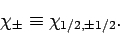(734)

It thus follows, from Eqs. (717) and (718), that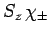(735)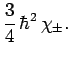(736)

Note thatcorresponds to an electron whose spin angular momentum vector has a positive component along the-axis. Loosely speaking, we could say that the spin vector points in the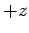-direction (or its spin is up''). Likewise,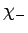corresponds to an electron whose spin points in the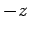-direction (or whose spin is down''). These two eigenstates satisfy the orthonormality requirements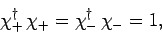(737)

and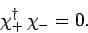(738)

A general spin state can be represented as a linear combination ofand: i.e.,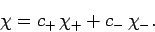(739)

It is thus evident that electron spin space is two-dimensional.

Up to now, we have discussed spin space in rather abstract terms. In the following, we shall describe a particular representation of electron spin space due to Pauli. This so-called Pauli representation allows us to visualize spin space, and also facilitates calculations involving spin.

Let us attempt to represent a general spin state as a complex column vector in some two-dimensional space: i.e.,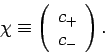(740)

The corresponding dual vector is represented as a row vector: i.e.,(741)

Furthermore, the productis obtained according to the ordinary rules of matrix multiplication: i.e.,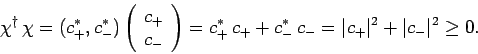(742)

Likewise, the product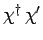of two different spin states is also obtained from the rules of matrix multiplication: i.e.,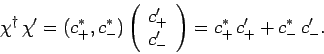(743)

Note that this particular representation of spin space is in complete accordance with the discussion in Sect. 10.3. For obvious reasons, a vector used to represent a spin state is generally known as spinor.

A general spin operatoris represented as a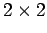matrix which operates on a spinor: i.e.,(744)

As is easily demonstrated, the Hermitian conjugate ofis represented by the transposed complex conjugate of the matrix used to represent: i.e.,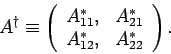(745)

Let us represent the spin eigenstatesandas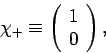(746)

and(747)

respectively. Note that these forms automatically satisfy the orthonormality constraints (737) and (738). It is convenient to write the spin operators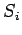(where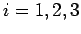corresponds to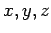) as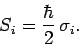(748)

Here, the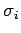are dimensionlessmatrices. According to Eqs. (702)-(704), thesatisfy the commutation relations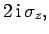(749)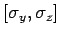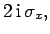(750)(751)

Furthermore, Eq. (735) yields(752)

It is easily demonstrated, from the above expressions, that theare represented by the following matrices: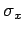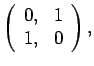(753)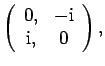(754)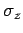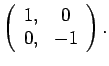(755)

Incidentally, these matrices are generally known as the Pauli matrices.

Finally, a general spinor takes the form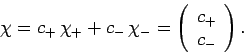(756)

If the spinor is properly normalized then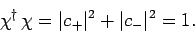(757)

In this case, we can interpret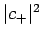as the probability that an observation of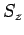will yield the result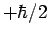, andas the probability that an observation ofwill yield the result.Next: Spin Precession Up: Spin Angular Momentum Previous: Eigenstates of and
Richard Fitzpatrick 2010-07-20